﻿ Metaanalysis of the Influence of Problem-Based Learning Model on Mathematic Problem Solving AbilityPublications are Open
Access in this journal
Article Versions
Export Article
• Normal Style
• MLA Style
• APA Style
• Chicago Style
Research Article
Open Access Peer-reviewed

### Metaanalysis of the Influence of Problem-Based Learning Model on Mathematic Problem Solving Ability

Swandi Wiranata Sinurat , E. Elvis Napitupulu, Mulyono
American Journal of Educational Research. 2021, 9(9), 566-570. DOI: 10.12691/education-9-9-2
Received July 23, 2021; Revised August 30, 2021; Accepted September 10, 2021

### Abstract

This study aims to determine: (1) Analyzing the effect size of the Problem-based Learning model on students' mathematical problem solving abilities; (2) To find out the meta-analysis of the effect of the problem-based learning model on students' mathematical problem solving abilities. Researchers meta-analyze journals about the effect of problem-based learning model on mathematical problem solving abilities with quantitative descriptive methods. The results showed that: (1) Of the 14 studies that met the criteria for a meta-analysis of the problem-based learning model in improving the mathematical problem solving ability of junior high school students, the highest effect size was 0.92; (2) The results of the meta-analysis of learning models on mathematical problem solving abilities are included in the large category with rRE = 0.53.

### 1. Introduction

Problem solving ability is an important component for learning mathematics. Mathematical problem solving is a focus in mathematics learning which includes closed problems with a single solution, open problems with non-single solutions and problems with various solutions (BSNP, 2006) 1. Training students with problem solving in learning mathematics is not just expecting to be able to solve the problems or problems given, but it is hoped that in carrying out the problem solving process, they are able to live a life full of complex problems.

Polya (1973:3) 2 defines problem solving as an effort to find a solution from a difficulty to achieve results that cannot be achieved. Problem solving is a psychological process that involves more than just learned propositions or theorems. A simple understanding of problem solving ability is the process of solving problems as a challenge to solve them. To be able to solve mathematical problems well, appropriate steps or problem-solving strategies are needed.

The facts show that the problem solving ability of students in Indonesia is still low. According to the results of the PISA survey in 2015 (OECD, 2016) 3, Indonesia was ranked 63 of the 72 participating countries with an average score of 386 for mathematics with an average international score of 490. Other references to Indonesian students' mathematics learning outcomes, especially about solving the mathematical problem is the result of an evaluation conducted by TIMSS. The international average for problem solving problems in geometry is 32%, the highest achievement achieved by Singapore students is 75%, while Indonesian students are only 19%. For problem solving in algebra, the international average is 18%, only 8% for Indonesian students.

According to Tan (Rusman, 2012) 4 Problem-based Learning is the use of various kinds of intelligence needed to confront real-world challenges and the ability to face all kinds of new things and existing complexities. Problem-based learning is a series of learning activities, meaning that in its implementation there are a number of activities that students must do. Problem-based learning does not expect students to just listen, take notes and then memorize the subject matter, but students actively think, communicate, search and process data and finally conclude. Problem-based learning activities are directed at solving problems and placing problems as keywords in the learning process (Rahman, 2018) 5.

To determine the effect of the Problem-based Learning model on students' mathematical problem solving abilities, several studies were carried out. Several studies by Fujasari, Handayani, Ubaidillah, and Shobrina used two learning models, namely the experimental class with the application of the Problem-based Learning model and the control class with conventional learning. The difference in mathematical problem solving ability between the control class and the experimental class is Fujasari of 16.51; Handayani of 8.26; Ubaidillah of 10.90; Shobrina of 6.7. The difference in students' mathematical problem solving abilities means that the application of the Problem-based Learning model can improve students' mathematical problem solving abilities when compared to conventional learning.

Based on the diversity of existing similar studies and the differences in the results of these studies, it is necessary to organize the data, dig up as much information as possible from the previous research obtained, and approach the comprehensiveness of the data with other purposes and there is no meta-analysis study in some of these experimental studies. Therefore, with previous research, it is necessary to re-analyze as a whole because a study generally has shortcomings or errors. There is a reality that in a study there is no research that is free from errors in research even though researchers have tried to minimize errors or errors in the study (Retnawati, 2018) 6.

Often research with the same case using the same method is carried out not only once, either by different researchers or by the same researcher, but at different times or by different samples. This results in different research results. Therefore, we need a combined research result that will be used as an inference on the parameters calculated in the study. The method used for this purpose is known as meta-analysis. In principle, meta-analysis is a way to obtain combined statistical inferences from research parameters based on the results of research that has been done.

According to Glass (1981) 7 meta-analysis is a quantitative analysis and uses a large amount of data and applies statistical methods by practicing it in organizing a number of information from large samples whose function is to complement other purposes. According to Borg (1983) 8 meta-analysis is a development technique to help researchers find consistency or inconsistency in cross-examination of the results of similar research. Sutjipto (1995) 9 defines meta-analysis as an attempt to summarize various research results quantitatively. In other words, meta-analysis as a technique is intended to re-analyze research results that are statistically processed based on primary data collection. Sugiyanto (2004) 10 argues that meta-analysis is a study by analyzing data from primary studies. The results of the primary study analysis are used as the basis for accepting or supporting the hypothesis and can also be used to reject/abort the hypothesis proposed by several researchers. It can be concluded that meta-analysis is a technique used to summarize various research results quantitatively by looking for effect size values. The effect size is searched by finding the difference between the experimental class average and the control class average, then dividing by the standard deviation of the control class. So from some of these definitions meta-analysis can be interpreted as data collection, processing and presentation activities that are carried out systematically and objectively to solve a problem or test a hypothesis by conducting an investigation of existing studies by describing and examining the parts of each research and the relationship of each research to obtain conclusions and in-depth understanding of the research studied. In other words, meta-analysis is a form of quantitative research that uses numbers and statistical methods from several research results to organize and extract as much information as possible from the data obtained, so as to approach comprehensiveness with other purposes.

### 2. Methods

The type of research used is descriptive quantitative research, namely research that is used to analyze data by describing or describing the data that has been collected as it is related to the effect of the Problem-based Learning model on the ability of mathematical problem solving data.

This research is a method obtained from the library by reading and studying journals about the effect of the Problem-based Learning learning model on students' mathematical problem solving abilities and self-efficacy. The subjects in this study were articles published in national and international journals about the effect of the Problem-based Learning learning model on students' mathematical problem solving abilities as many as 43 journals to be selected. screening of articles about the effect of the Problem-based Learning model on junior high school students. Then after being filtered based on the effect of the Problem-based Learning model on the mathematical problem solving ability of SMP there were 15 articles that were suitable and there were 3 journals that were not verified. The articles contained are the results of research conducted by researchers at the junior high and high school levels. While the object in this study is the effect of the Problem-based Learning model on students' mathematical problem solving abilities, with the following types of research categories, namely:

1. The research was conducted in journals that have been published nationally and internationally, and have been accredited by the Ministry of Research, Technology and Higher Education of the Republic of Indonesia (Ristekdikti) to Sinta.

2. The article is a quantitative study and meets the effect size data.

3. Articles with the theme of Problem-based Learning models on students' mathematical problem solving abilities

4. Articles published in the last ten years

5. Educational levels in journals are all levels of education with the scope of the research area being carried out in the Indonesian region.

The steps for analyzing data with meta-analysis are as follows:

1. Identify the mean and standard deviation of each research article, written based on the results in the experimental class and control class.

2. Calculating effect size

The effect size formula used is the eta square formulaKadir (2014: 300) 11 says that experimental research that only involves two control groups, namely the experimental group of two control groups uses comparative analysis with the t-test analysis technique, then uses the effect size formula as follows:For experimental research involving more than two groups using comparative analysis with the 1-way ANOVA analysis technique with the following formula: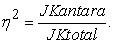For experimental research involving more than two groups and their interactions, using comparative analysis with the Anova – 2 way analysis technique, so the formula used is: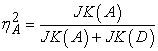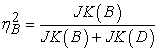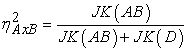Experimental research with the assumption of a heterogeneous group with the assumption of a heterogeneous group two groups of formulas are used (Glass, 2012) 12: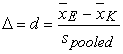When,for sample data. The criteria used in forming the interpretation of the results of the effect size correlation use the reference from Cohen (1997) 13 namely: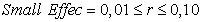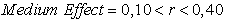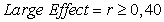3. Looking for Standart Error

To find the standard error in meta-analysis research, you can use the following formula:Calm:

SE: Standart Error

ES: Effect Size

4. Test for heterogeneity, Summary Effect, bias correction with software JASP

### 3. Results and Discussion

Data from Classical Meta-analysis of PBL Learning Models to Improve Mathematical Problem Solving Ability of Junior High School Students by Using JASP software

The following is a summary of the data resulting from the effect size and standard error of increasing mathematical problem solving abilities of junior high school students can be seen in Table 1.

Heterogeneity Test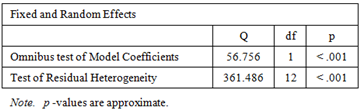From the table above, it can be seen that the number listed in df is 13, meaning that the effect size results analyzed in this study were 14 effect sizes. The results of the analysis of the JASP software on 14 effect sizes from several articles showed heterogeneity. This can be seen from the "Test of Residual Heterogeneity" which has p < .001 (Q = 424.298; p < .001) which means it is significant. These results indicate that there is an effect of the PBL learning model on the mathematical problem solving ability of junior high school students.

Summary effect/ Mean Effect Size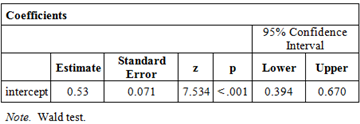From the table, it can be seen that there is a significant positive correlation between the PBL learning model and the mathematical problem solving abilities of junior high school students (z = 7,534; p < 0.001; 95% CI [0.394; 0.670]). The mean effect size of the influence of the PBL learning model on the mathematical problem solving ability of junior high school students is included in the large category with rRE= 0.511.

Forest PlotFrom the Forest plot, it can be observed that the effect size of the analyzed articles varies from 0.17 to 0.92 with an average of 0.53. In figure above it can be seen that in each article there is a stem in the forest plot that determines the value of the range, its average and also its significance. The bar limit shown is the range of the upper and lower limit intervals at a 95% confidence interval. While the black square shape is the level of significance. The wider the square shape, the greater the significance. Vice versa. In figure above, it can be seen that KPM 13 is the most significant data among all the effect size values.

Evaluation of Publication Bias

To see publication bias in meta-analysis research can use funnel plots, Egger's test, and Fail-Safe N.

a) Funnel Plot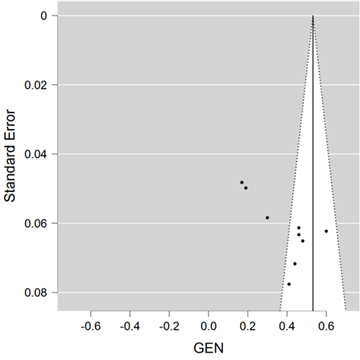From the figure above, it is difficult to conclude whether the funnel plot is symmetrical or not, so an egger's test is needed to test whether the funnel plot is symmetrical or not.

b) Egger's test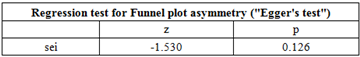Based on the data above shows p > 0.05 which confirms that the funnel plot is symmetrical. Thus, it can be concluded that there is no publication bias problem in the meta-analysis studies conducted.

c) Fail-Safe NFrom Rosenthal's assumption there is a 5k+10 formula for the minimum value of publication bias, where k is the number of studies. If the value of Fail-safe N is 6572,000 > 5k+10, it means that there is no problem with publication bias in meta-analytical studies. Because there are 13 meta-analytical studies, 5(13) + 10 = 80 and 6572,000 > 80. So it can be concluded that there is no problem of publication bias in the meta-analysis studies.

From the three proofs of publication bias, it can be concluded that there is no problem with publication bias in the meta-analysis study of the PBL learning model on the mathematical problem solving ability of junior high school students.

The results of this study obtained the effect size of the influence of the PBL learning model on the mathematical problem solving ability of junior high school students including in the large category with rRE = 0.53. it can be concluded that the PBL learning model on students' mathematical problem solving abilities has a great influence. This is in line with the research conducted by Bettri Yustinaningrum (2021) entitled Meta Analysis: The Effect of Problem Based Learning Models on Mathematical Problem Solving Ability which obtained an average effect size of 2.02 with a very large category. Meanwhile, based on the paired sample t-test, the value of sig (2 tailed) = 0.000 < = 0.05, which means that there is a difference in the average problem-solving ability before and after being treated with the problem-based learning model. Based on the average effect size and the results of the paired sample t-test, it can be concluded that the problem-based learning model has an effect on students' mathematical problem solving abilities.

Another study by Umaroh and M. Zainudin entitled Meta-Analysis Study: The Effect of Problem Based Learning (PBL) on Students' Mathematical Problem Solving Ability (2020) also showed that the effect size results based on the overall category obtained an average effect size of 12 articles of 0.242 ( medium category) with four articles in the small category, five articles in the medium category, and three articles in the large category. Based on the level of education, it was found that the high school level had a larger effect size than the junior high school level, namely 0.363 (large category). For the location of the region, the island of Sumatra obtained the highest effect size results than the islands of Java and Bali with a large influence of 0.209 (medium category). Meanwhile, based on the dependent variable, this study obtained the results that learning with the Problem Based Learning (PBL) model on mathematical problem solving ability has an effect size of 0.242, learning independence of 0.134, and mathematical confidence of 0.142. So it can be concluded that learning using the Problem Based Learning (PBL) model has a major influence on students' mathematical problem solving abilities.

### 4. Conclusions

Of the 13 studies that meet the criteria for a meta-analysis of problem-based learning models in improving mathematical problem solving abilities of junior high school students, the research conducted by Sitti Rahmah Tahir with a model with problem-based learning in improving mathematical problem solving skills is the article that has the highest effect size. with a value of 0.92 among others. Of the 9 studies that meet the criteria for a meta-analysis of problem-based learning models in improving self-efficacy of junior high school students, the research conducted by Catur Budi Nugroho, Fahinu and Asrul Sani with problem-based learning models in increasing self-efficacy is an article that has an effect the highest size with a value of 0.88 among others. Based on the results of the meta-analysis that has been carried out, it is found that with the random effects model there is a significant positive correlation between problem-based learning models and students' mathematical problem solving abilities. The influence of the learning model on mathematical problem solving abilities is included in the large category with rRE = 0.511.

### References

  BSNP. (2006). Panduan Penyusunan Kurikulum Tingkat Satuan Pendidikan Jenjang Pendidikan Dasar dan Menengah. Jakarta: Depdiknas RI. In article  Polya, G. 1973. How to Solve It. New York: Doubleday. In article  OECD Publishing. (2016). PISA 2015 Result. OECD Publishing. In article  Rusman. (2012). Model-Model Pembelajaran: Mengembangkan Professionalisme Guru. Jakarta: Rajagrafindo Persada. In article  Rahman, A.A. (2018). Strategi Belajar Mengajar Matematika. Banda Aceh: Syiah Kuala University Press. In article  Retnawati, H. (2018). Penghantar Meta Analisis. Yogyakarta: Parama Publishing.Rusman. (2012). Model-Model Pembelajaran: Mengembangkan Professionalisme Guru. Jakarta: Rajagrafindo Persada. In article  Glass, G.V., Mcgaw B., & Smith, M.L. (1981). Meta-Analysis in Social Research. London: Sage Publication. In article  Borg, W.R & Gall, M.D. Gall. (1983). Educational Research: An Introduction, Fifth Edition, New York: Longman. In article  Sutjipto, H.P. (1995). Aplikasi Meta-Analisis dalam Pengujian Validitas Aitem. Buletin Psikologi, 3(2): 20-28. In article  Sugiyanto. 2004. Perpustakaan Sekolah. Jakarta: Kompas. www.kompas .com diakses pada 14 Maret 2021. In article  Kadir, (2014). Meta-analysis of the Effect of Learning Interventation Toward Mathemacal Thinking on Research and Publication of Students, Journal of Education in Muslim Society, Vol.4. No.2. In article View Article  Glass, G. (2012). Meta-Analysis of Research on Class Size and Achievment. Jstore. Vol.1. No. 1. In article View Article  Cohen, J. (1997). Statistical power analysis for the behavioral sciences. New NY: Academic Press. In article

Published with license by Science and Education Publishing, Copyright © 2021 Swandi Wiranata Sinurat, E. Elvis Napitupulu and MulyonoThis work is licensed under a Creative Commons Attribution 4.0 International License. To view a copy of this license, visit http://creativecommons.org/licenses/by/4.0/